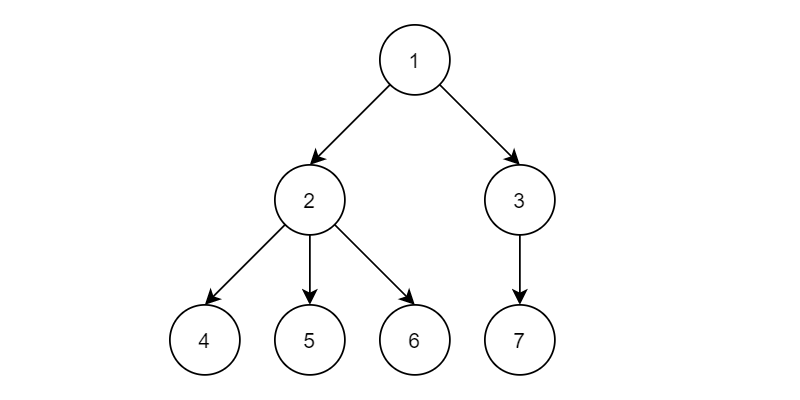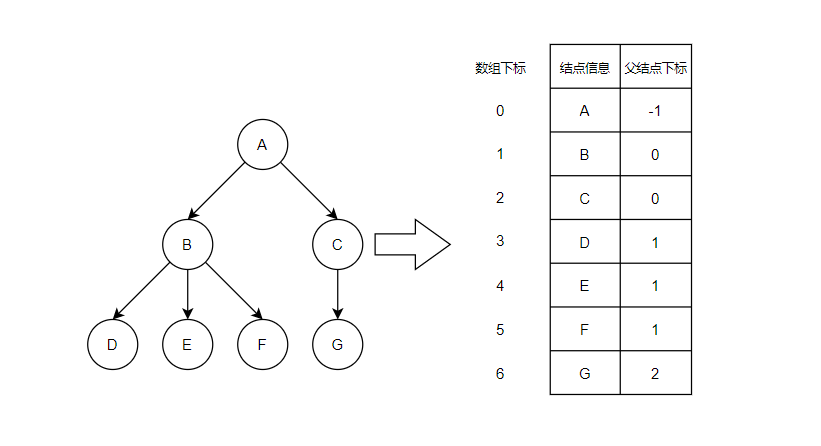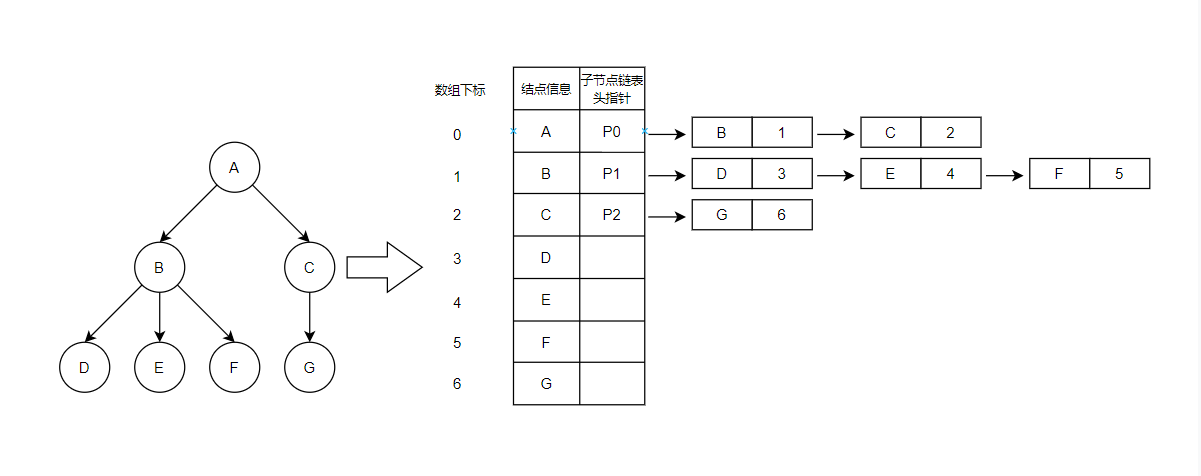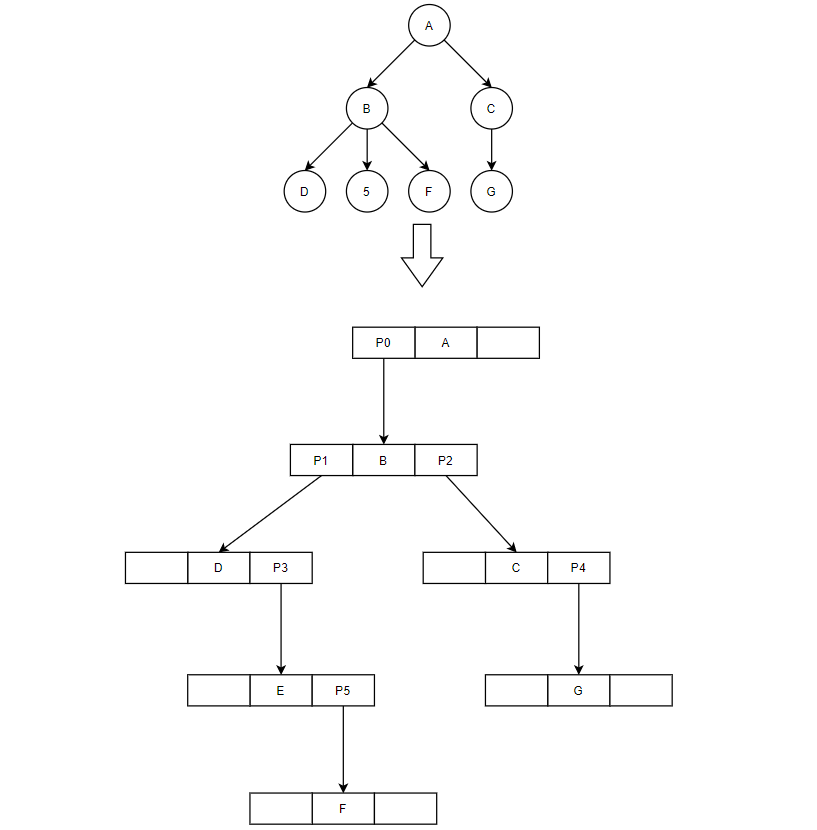# 概念

• 每个结点都只有有限个子结点或无子结点
• 没有父结点的结点称为根结点
• 每一个非根结点有且只有一个父结点
• 除了根结点外，每个子结点可以分为多个不相交的子树
• 树里面没有环路(cycle)

# 相关术语

• 结点
树中的一个独立单元，之前我们说了，树是一个集合，结点就是这个集合中的一个元素。
• 结点的度
结点拥有的子树数称为结点的度。
• 树的度
树的度是树内各结点度的最大值。
• 叶子（叶子结点）
度为 0 的结点称为叶子或终端结点。
• 非终端结点（分支结点）
度不为 0 的结点称为非终端结点或分支结点。除根结点之外，非终端结点也称为内部结点。
• 双亲（双亲结点）、孩子（孩子结点）
结点的子树的根称为该结点的孩子，相应地，该结点称为孩子的双亲。
• 兄弟（结点）
同一个双亲的孩子之间互称兄弟。
• 祖先（祖先结点）
从根到该结点所经分支上的所有结点。
• 子孙（子孙结点）
以某结点为根的子树中的任一结点都称为该结点的子孙。
• 层次
结点的层次从根开始定义起，根为第一层，根的孩子为第二层。树中任一结点的层次等千其双亲结点的层次加 1。
• 堂兄弟（堂兄弟结点）
双亲在同一层的结点互为堂兄弟。
• 树的深度、树的高度
树的深度或高度数值上等于树中结点的最大层次，深度以根结点为 1 向叶子结点递增，高度以叶子结点为 1 向根结点递增。
• 结点的深度、结点的高度
结点的深度或高度数值上等于以该结点为根结点的子树的最大层次，深度以目标结点为 1 向叶子结点递增，高度以叶子结点为 1 向目标结点递增。
• 有序树和无序树
如果将树中结点的各子树看成从左至右是有次序的（即不能互换），则称该树为有序树，否则称为无序树。在有序树中最左边的子树的根称为第一个孩子，最右边的称为最后一个孩子。
• 森林
m (m≥0)棵互不相交的树的集合。
• 路径
两个结点之间经过的结点序列，路径的方向为子结点指向子结点（自上而下）。
• 路径长度
一条路径中经过的边的条数。
• n 叉树
一棵树中允许结点拥有子树的最大数目，如二叉树允许一个结点最多可拥有两个子树。

# 形象描述# 树的存储结构

## 双亲表示法## 孩子表示法## 孩子兄弟表示法# 树的相关推论

## 结点拥有的结点数为所有结点的度数+1

### 证明：

由结点的度和孩子结点的概念可得，结点的度数=该结点孩子结点的个数

∵ 除根结点外其余结点都可以作为孩子结点

∴ 树的结点总数=孩子结点总数+根结点数=所有结点的度数+根结点数=所有结点的度数+1

## 一棵有 n 个结点的树有 n-1 条边（结点之间的连线）

### 证明：

∵ 树中除根结点外每个结点都有一个父结点，即这些结点都有一条线与父结点连接

∴ 边的数目为结点数减去根结点数 1

## 度为 m 的树中第 i 层最多有 mi-1个结点（i≥1）

### 证明：

若考虑最多的情况，则每一个结点的度都为 m，即每一个结点都有 m 个孩子结点，即第 i 层的每一个结点在第 i+1 层都会产生 m 个孩子结点。

设N(i)为第 i 层的结点数，第 i+1 层结点数可表示为 N(i)×m

∵N(i)的个数为N(i-1)×m，可得 N(i+1)=N(i-1)×m×m

依次进行如上的转化，可得到N(i+1)=N(i-n)×mn+1

即 N(i)=N(i-n)×mn

这是一个通式，知道任意一层结点的个数即可推出其他层结点的个数，最好考虑的是第一层

∵ N(1)=1

∴ 将上面的 i-n 化为 1

可得N(i)=N(1)×mi-1

∴ 度为 m 的树中第 i 层最多有mi-1个结点

## 高度为 h 的 m 叉树至多有 (mh-1)÷(m-1)个结点

### 证明：

考虑最多情况，m 叉树的每一个结点都会在下一层产生 m 个结点

设N(h)为第 h 层的结点数，第 h+1 层结点数可表示为 N(h)×m

设数列{ah}，a1=N(1)，a2=N(2)，ah=N(h)，ah+1=N(h+1) (h≥1)

由条件可知a1=N(1)=1

∵ah+1÷ah=N(h+1)÷N(h)=m，h≥2

根据定义，数列{ah}是以首项为1，公比为m(m>0)的等比数列

设数列{ah}的前n项和为Sh

由求和公式

$$S_n=\frac{a_1\times(1-q^n)}{1-q}, (q\neq1)$$

a1=1，q=m，m≠1，n=h

可得

$$S_n=\frac{(1-m^h)}{1-m}$$

## 具有 n 个结点的满 m 叉树的最小高度为 logm(n(m-1)+1)

### 证明：

$$S_h=\frac{(1-m^h)}{1-m}$$

$$h=\log_m{(n(m-1)+1)}$$